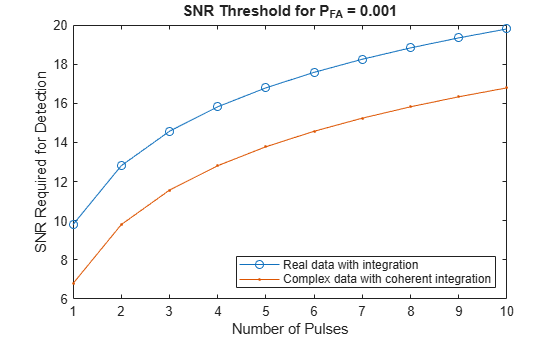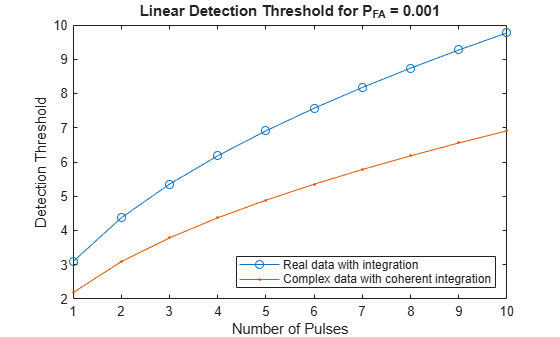# npwgnthresh

Detection SNR threshold for signal in white Gaussian noise

## Syntax

``snrthresh = npwgnthresh(pfa)``
``snrthresh = npwgnthresh(pfa,numpulses)``
``snrthresh = npwgnthresh(pfa,numpulses,dettype)``
``snrthresh = npwgnthresh(pfa,numpulses,dettype,outscale)``

## Description

````snrthresh = npwgnthresh(pfa)` calculates the Detection SNR Threshold in decibels for detecting a deterministic signal in white Gaussian noise. The detection uses the Neyman-Pearson (NP) decision rule to achieve a specified probability of false alarm, `pfa`. This function uses a square-law detector.```

````snrthresh = npwgnthresh(pfa,numpulses)` specifies `numpulses` as the number of pulses used in the pulse integration.```

````snrthresh = npwgnthresh(pfa,numpulses,dettype)` specifies `dettype` as the type of detection. A square law detector is used in noncoherent detection.```

````snrthresh = npwgnthresh(pfa,numpulses,dettype,outscale)` specifies the output scale.```

## Examples

collapse all

Calculate the detection threshold that achieves a probability of false alarm (pfa) of 0.01. Assume a single pulse with a `real` detection type. Then, verify that this threshold produces a pfa of approximately 0.01. Do this by constructing 10000 real white gaussian noise (wgn) samples and computing the fraction of samples exceeding the threshold.

Compute the threshold from pfa. The detection threshold is expressed as a signal-to-noise ratio in db.

```pfa = 0.01; numpulses = 1; snrthreshold = npwgnthresh(pfa,numpulses,"real")```
```snrthreshold = 7.3335 ```

Compute fraction of simulated noise samples exceeding the threshold. The noise has unit power with 10000 samples.

```noisepower = 1; Ntrial = 10000; noise = sqrt(noisepower)*randn(1,Ntrial);```

Express the threshold in amplitude units.

```threshold = sqrt(noisepower*db2pow(snrthreshold)); calculated_Pfa = sum(noise>threshold)/Ntrial```
```calculated_Pfa = 0.0107 ```

Plot the SNR detection threshold against the number of pulses, for real and complex noise. In each case, the SNR detection threshold is set for a probability of false alarm (pfa) of 0.001.

Compute detection threshold for 1 to 10 pulses of real and complex noise.

```Npulses = 10; snrcoh = zeros(1,Npulses); snrreal = zeros(1,Npulses); Pfa = 1e-3; for num = 1:Npulses snrreal(num) = npwgnthresh(Pfa,num,"real"); snrcoh(num) = npwgnthresh(Pfa,num,"coherent"); end```

Plot the detection thresholds against number of pulses.

```plot(snrreal,"o-") hold on plot(snrcoh,".-") hold off legend("Real data with integration",... "Complex data with coherent integration",... Location="southeast") xlabel("Number of Pulses") ylabel("SNR Required for Detection") title("SNR Threshold for P_F_A = "+Pfa)```Plot the linear detection threshold against the number of pulses, for real and complex data. In each case, the threshold is set for a probability of false alarm of 0.001.

Compute detection threshold for 1 to 10 pulses of real and complex noise.

```Npulses = 10; snrcoh = zeros(1,Npulses); snrreal = zeros(1,Npulses); Pfa = 1e-3; for num = 1:Npulses snrreal(num) = npwgnthresh(Pfa,num,"real","linear"); snrcoh(num) = npwgnthresh(Pfa,num,"coherent","linear"); end```

Plot the detection thresholds against number of pulses.

```plot(snrreal,"o-") hold on plot(snrcoh,".-") hold off legend("Real data with integration",... "Complex data with coherent integration",... Location="southeast") xlabel("Number of Pulses") ylabel("Detection Threshold") title("Linear Detection Threshold for P_F_A = "+Pfa)```## Input Arguments

collapse all

Probability of false alarm, specified as a scalar in the range `(0, 1)`.

Data Types: `double`

Number of pulses used in the integration, specified as a positive integer.

Data Types: `double`

Specifies the type of pulse integration used in the NP decision rule, specified as `"coherent"`, `"noncoherent"`, or `"real"`. `"coherent"` uses magnitude and phase information of complex-valued samples. `"noncoherent"` uses squared magnitudes. `"real"` uses real-valued samples.

Data Types: `char` | `string`

Scale of the output value, specified as `"db"` or `"linear"`. When `outscale` is set to `"linear"`, the returned threshold represents amplitude.

Data Types: `char` | `string`

## Output Arguments

collapse all

Detection threshold, returned as a scalar. The detection threshold is expressed in terms of the signal-to-noise ratio in decibels or in linear units if `outscale` is set to `"linear"`. The relationship between the linear threshold and the threshold in dB is

`${T}_{dB}=20{\mathrm{log}}_{10}{T}_{lin}$`

collapse all

### Detection SNR Threshold

The output of `npwgnthresh` determines the detection threshold required to achieve a particular Pfa.

The threshold increases when pulse integration is used in the receiver. This threshold is not the single sample SNR that is used as an input to `rocsnr` or as the output of `rocpfa`, `albersheim`, and `shnidman`. For any fixed Pfa, you can decrease the single sample SNR required to achieve a particular Pd when pulse integration is used in the receiver. See Signal Detection in White Gaussian Noise and Source Localization Using Generalized Cross Correlation for examples of how to use `npwgnthresh` in a detection system.

### Detection in Real-Valued White Gaussian Noise

This function is designed for the detection of a nonzero mean in a sequence of Gaussian random variables. The function assumes that the random variables are independent and identically distributed, with zero mean. The linear detection threshold λ for an NP detector is

`$\frac{\lambda }{\sigma }=\sqrt{2N}\text{\hspace{0.17em}}{\text{erfc}}^{-1}\left(2{P}_{fa}\right)$`

This threshold can also be expressed as a signal-to-noise ratio in decibels

`$10{\mathrm{log}}_{10}\left(\frac{{\lambda }^{2}}{{\sigma }^{2}}\right)=10{\mathrm{log}}_{10}\left(2N{\left({\text{erfc}}^{-1}\left(2{P}_{fa}\right)\right)}^{2}\right)$`

In these equations

• σ2 is the variance of the white Gaussian noise sequence

• N is the number of samples

• erfc—1 is the inverse of the complementary error function

• Pfa is the probability of false alarm

Note

For probabilities of false alarm greater than or equal to 1/2, the formula for detection threshold as SNR is invalid because erfc-1 is less than or equal to zero for values of its argument greater than or equal to one. In that case, use the linear output of the function invoked by setting `outscale` to`'linear'`.

### Detection in Complex-Valued White Gaussian Noise (Coherent Samples)

The NP detector for complex-valued signals is similar to that discussed in Source Localization Using Generalized Cross Correlation. In addition, the function makes these assumptions:

• The variance of the complex-valued Gaussian random variable is divided equally among the real and imaginary parts.

• The real and imaginary parts are uncorrelated.

Under these assumptions, the linear detection threshold for an NP detector is

`$\frac{\lambda }{\sigma }=\sqrt{N}\text{\hspace{0.17em}}{\text{erfc}}^{-1}\left(2{P}_{fa}\right)$`

and expressed as a signal-to-noise ratio in decibels is:

`$10{\mathrm{log}}_{10}\left(\frac{{\lambda }^{2}}{{\sigma }^{2}}\right)=10{\mathrm{log}}_{10}\left(N{\left({\text{erfc}}^{-1}\left(2{P}_{fa}\right)\right)}^{2}\right)$`

Note

For probabilities of false alarm greater than or equal to 1/2, the formula for detection threshold as SNR is invalid because erfc-1 is less than or equal to zero for when its argument is greater than or equal to one. In that case, select linear output for the function by setting `outscale` to `'linear'`.

### Detection of Noncoherent Samples in White Gaussian Noise

For noncoherent samples in white Gaussian noise, detection of a nonzero mean leads to a square-law detector. For a detailed derivation, see , pp. 324–329.

The linear detection threshold for the noncoherent NP detector is:

`$\frac{\lambda }{\sigma }=\sqrt{{P}^{-1}\left(N,1-{P}_{fa}\right)}$`

The threshold expressed as a signal-to-noise ratio in decibels is:

`$10{\mathrm{log}}_{10}\left(\frac{{\lambda }^{2}}{{\sigma }^{2}}\right)=10{\mathrm{log}}_{10}{P}^{-1}\left(N,1-{P}_{fa}\right)$`

where ${P}^{-1}\left(x,y\right)$is the inverse of the lower incomplete gamma function, Pfa is the probability of false alarm, and N is the number of pulses.

 Kay, S. M. Fundamentals of Statistical Signal Processing: Detection Theory. Upper Saddle River, NJ: Prentice Hall, 1998.

 Richards, M. A. Fundamentals of Radar Signal Processing. New York: McGraw-Hill, 2005.